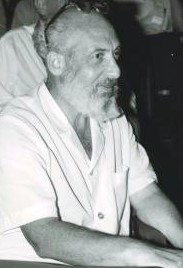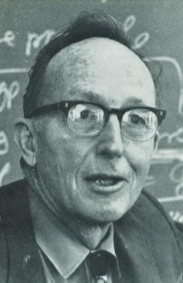### Contravariance and Covariance - Part 5Samuel EilenbergSaunders Mac Lane

Category Theory
Category Theory was developed by Eilenberg and MacLane  in algebraic topology. In a heuristic sense, it may be considered as the “mathematics of mathematics”. Different branches of mathematics formally share structural similarities, which category theory attempts to study.
As an example of how different mathematical fields can have a similar structure, consider the mathematical subjects of topology, linear algebra, group theory, and differential geometry.
Given a collection of open sets that form a topology, one of the questions topologists are interested in is what maps preserve the topological structure of the topology. Such functions turn out to be the homeomorphisms. In linear algebra, we are interested in those maps which preserve the vector space structure. These maps turn out to be the isomorphisms. In group theory, we are interested in maps which preserve the group structure. These maps turn out to be the homomorphisms. In differential geometry, we are interested in those maps which preserve the differential structure of smooth manifolds, and these turn out to be the diffeomorphisms.
It is evident even to the casual observer that there is a commonality between the above examples. In general, we have a set of objects, and maps defined on those objects, such that the maps preserve the mathematical structure of the objects. Category theory, among other things, abstracts this commonality and makes it precise. This generality of category theory means that it can be possible to prove a theorem in general categorical terms, and possibly have it apply to all categories.
Now, on to the definition of a category. But first, a little set theory trivia. In order to avoid any paradoxes that can arise from considering sets of sets, such as Russell’s Paradox, mathematicians will sometimes circumvent the issue by talking about “classes” of sets instead. That is why the definition given below defines a category in terms of a class of sets, and not as a set of sets.
##### Category
A category is a class C of objects such that for any two objects A and B  in C, there is a class of disjoint sets, Mor(A,B), the elements of which are called morphisms from A to B. If f is a morphism from A to B, it is denoted by f : A →B.
The following properties are required to hold:

a) Composition: For any three objects A,B and C, if f : A →B is a morphism from A to B, and g : B →C is a morphism from B to C, then there exists a morphism from A to C, denoted by g ◦ f and called the composite of f with g.

b) Associativity: Composition of morphisms is required to satisfy the associative law:  f ◦ (h ◦ g) = (f ◦ h) ◦ g

c) Identity: For any object A of C, there exits a morphism 1A : A →A such that if f : A → B is a morphism from A to B, then f ◦ 1A = f and if g : C → A is a morphism from C to A, then 1A ◦ g = g

Examples of categories, as already alluded to, are the category of topological spaces, the category of vector spaces, the category of groups, and the category of smooth manifolds.
Once again, the power of the idea is the following...it might be possible to prove a single theorem in category theory, and if it turns out the categorical conditions of the proof were present in the category of groups and the category of vector spaces, then as a special case, you have also just proved theorems in group theory and in linear algebra. Not too bad.
But wait, as they say, there is more. Not just proofs, but mathematical constructions and definitions can be formulated in general categorical terms, which can be very helpful.
So, for example, say you are a topologist, and suppose you were not sure of how you should define the product topology of two topological spaces A and B. There are in fact a couple of plausible definitions. The most obvious definition is the box topology, but it turns out the box topology is not the topology mathematicians generally use for products. The box topology works OK for finite products of topological spaces, but for arbitray products (i.e., infinite), it does not. Now, if you have in your toolbox the general categorical notion of a product, then you are more likely to discover the actual, correct for infinite products, product topology ... Again, not too bad.

##### Covariant Functor
Let C and D be categories. A covariant functor from C to D consists of two functions, an object function that maps objects in C to objects in D, and a morphism function that maps morphisms in C to morphisms in D. By convention, the same symbol F is used to designate both functions.

So if f ∈ Mor(A,B) is a morphism in C, then F(f) ∈ Mor(F(A), F(B)) is a morphism in D. F is also required to satisfy:

i) if φ ∈ Mor(A,B) and ψ ∈ Mor(B,C) are morphisms in C, then
F(φ ◦ ψ) = F(φ) ◦ F(ψ)

ii) F(1A) = 1F(A) , for any object A in C

By making a minor change to the composition rule in (i) and also changing the direction of the morphism F(f) (i.e., reverse the arrow), we get the definition of a contravariant functor:
##### Contravariant Functor
Let C and D be categories. A contravariant functor from C to D consists of two functions, an object function that maps objects in C to objects in D, and a morphism function that maps morphisms in C to morphisms in D. By convention, the same symbol F is used to designate both functions.

So if f ∈ Mor(A,B) is a morphism in C, then F(f) ∈ Mor(F(B), F(A)) is a morphism in D. F is also required to satisfy:

i) if φ ∈ Mor(A,B) and ψ ∈ Mor(B,C) are morphisms in C, then
F(φ ◦ ψ) = F(ψ) ◦ F(φ)

ii) F(1A) = 1F(A) , for any object A in C

References:

 S. Eilenberg and S. MacLane, General Theory of Natural Equivalences. Trans. Am. Math. Soc. 58, 231-294 (1945). 1968.